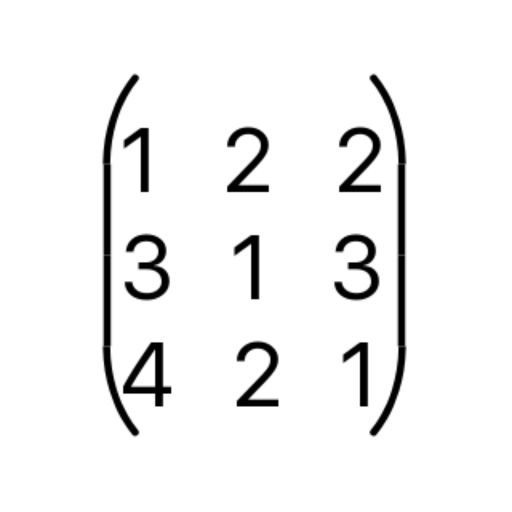Calculum# Calculum

## Peter Bohl

1.23 for iPhone, iPad
\$0.99
0

0 Ratings

Release Date

2017-07-25

Size

19.8 MB

### Screenshots for iPhone

iPhone
More Screenshots for iPad
Calculum Description
Calculum is the perfect support for mathematical tasks with matrices and vectors. Calculum has no limitation on the matrix and vector dimensions.
You want to solve a linear equation system?
With a wipe you have created the equation system and filled with values!
Do you need to add, subtract, or multiply vectors?
Simply enter and calculate the vectors using Calculum!
You have to create an equation system in triangle form?
A button and you have the finished result!
You have to calculate an inverse matrix?
No problem, Calculum takes this calculation quite simply the scare!
Matrix determinants?
No problem for Calculum!

Calculum is an indispensable tool for all students.

List of Functions:
- Solve linear equation systems
- Calculating the triangular form of matrices
- Matrix inversion
- Calculate the determinant of a matrix
- Calculate the inverse Matrix
- Add, subtract and multiply Matrices and/or Vectors
Calculum 1.23 Update
2017-07-25 Version History
Improved results display
More
Price:
\$0.99
Version:
1.23
Size:
19.8 MB
Release Date:
2017-07-25
Developer:
Peter Bohl
Language:
Chinese English French German Portuguese Russian Spanish
More
You May Also Like
Developer Apps